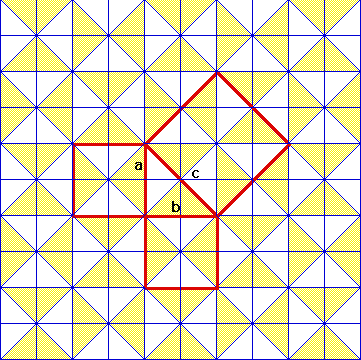# A Babylonian Tile Proof of the Pythagorean TheoremIf you count the triangles in squares a and b, on the legs of the right triangle, you will see that there are 8 in each. The square on the hypotenuse of the triangle, c, contains 16 triangles. So, the sum of the squares on the legs of the right triangle is equal to the square on the hypotenuse, as 8 + 8 = 16. It is thought that the Babylonians saw this pattern of tiles to be a proof of the Pythagorean Theorem.